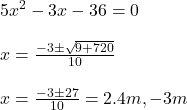## a rectangular lawn of dimensions 6x metres by x metres. In the centre is a rectangular flower bed of length (x + 4) m and width (x – 1) m.

Question

a rectangular lawn of dimensions 6x metres by x metres. In the centre is a rectangular flower bed of length (x + 4) m and width (x – 1) m. If the area of the shaded region is 40 m’, calculate the area of the flower bed.​

in progress 0
6 months 2021-08-26T04:56:35+00:00 1 Answers 2 views 0

## Answers ( )

The area of flower bed is 8.96 m^2.

Step-by-step explanation:

Length of lawn = 6 x

width of lawn = x

length of flower bed = x + 4

width of flower bed = x – 1

Area of shaded region = 40 m^2

Area of the shaded region

6 x (x) – (x +4)(x -1) = 40As length cannot have negative value, so x = 2.4 m.

Area of flower bed = (x + 4)(x – 1) = (2.4 + 4)(2.4 – 1) = 8.96 m^2